# What Is The Quadratic Formula? (3 Key Things To Know)

The quadratic formula is just one of many ways to solve a quadratic equation.  This method is a general one that will work for any quadratic equation we can think of.

So, what is the quadratic formula?  The quadratic formula gives us the solutions for a quadratic equation in the standard form ax2 + bx + c = 0. The formula is x = [-b +/- √(b2 – 4ac)] / 2a. The sign of the discriminant, b2 – 4ac, determines the solutions (two distinct real, one real repeated, or two complex conjugate solutions).

Of course, we can also use the quadratic formula to factor a quadratic.  In some cases, we can use the quadratic formula to solve quartic or higher order polynomial equations!

In this article, we’ll talk about the quadratic formula, when to use it, and how to use it, along with examples.  We’ll also answer a few common questions about the quadratic formula.

Let’s get started.

## What Is The Quadratic Formula?

The quadratic formula gives us an easy way to solve a quadratic equation in standard form.  No matter which quadratic equation we have, the quadratic formula can find a solution.

Remember that standard form for a quadratic equation looks like this:

• ax2 + bx + c = 0  [quadratic standard form]

where a is not zero.

When Does A Quadratic Have No Solut...
When Does A Quadratic Have No Solution?

The quadratic formula is given by: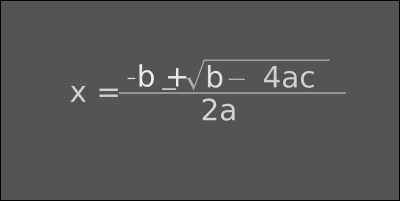The quadratic formula is a general way to solve any quadratic equation by finding the roots (zeros) of the equation.

Note that the solutions we find are the x-values that satisfy the equation ax2 + bx + c = 0.

Also, note that we will get two distinct solutions except in the case when the discriminant is zero (that is, when b2 – 4ac = 0, or b2 = 4ac).

The sign of the discriminant, b2 – 4ac, determines the type of solutions we get, based on the following three possible cases:

• Positive Discriminant (b2 – 4ac > 0): Two distinct real solutions
• Zero Discriminant (b2 – 4ac = 0): One repeated real solution
• Negative Discriminant (b2 – 4ac < 0): Two complex conjugate solutions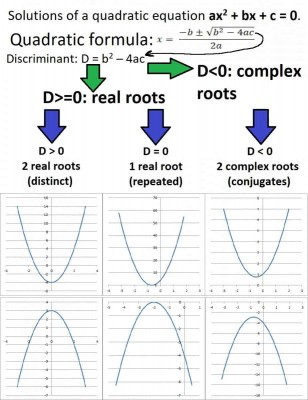The discriminant of a quadratic tells us the nature of the solutions. It also gives us an idea of what the graph (a parabola) looks like.

Remember that in the third case, our complex conjugates have the form r + si and r – si (here, s is not zero, so the two complex conjugate solutions are distinct).

### When To Use The Quadratic Formula

There are many scenarios when you might want to use the quadratic formula:

• Solving a polynomial equation of degree 2.  After combining like terms and making one side of the equation zero (quadratic standard form), we can solve using the quadratic formula.
• Locating the zeros (x-intercepts) of a parabola.  This is helpful to find 1 or 2 “base points” to graph when sketching a parabola (unless the solutions are complex conjugates).
• Finding the roots of a quadratic equation.  This is helpful when factoring is difficult.
• Dealing with gravity and falling objects in physics problems.

### How To Use The Quadratic Formula (Solving Quadratic Equations)

Using the quadratic formula is straightforward with a little practice if you follow the steps:

• First, get the equation into quadratic standard form, ax2 + bx + c = 0.  You may need to rearrange terms and combine like terms to do so.
• Next, identify the values of a, b, and c.  Sometimes it helps to write them somewhere to avoid missing any negative signs.  For example: a = 2, b = -3, c = 4.
• Then, write out the general quadratic formula, without any values plugged in.
• Now, rewrite the quadratic formula with the values of a, b, and c substituted.
• Finally, work to simplify the values in the formula until we cannot reduce any further.

Let’s look at some examples to see how the quadratic formula works in 3 different cases.  We’ll start with 2 distinct real solutions (a positive discriminant).

#### Example 1: Two Distinct Real Solutions (Positive Discriminant)

Consider the quadratic equation x2 – 3x + 2 = 0.  This equation is already in quadratic standard form.

We have coefficient values of a = 1, b = -3, and c = 2.

Remember that the quadratic formula is

Substituting into the quadratic formula, we get

After simplifying, we can reduce this to (3 +/- 1) / 2, or x = 2 and x = 1.  This quadratic equation has two distinct real solutions (which happen to be positive integers).

The graph of this quadratic (a parabola) has zeros at x = 1 and x = 2, with a vertex at x = 1.5 (halfway between the two zeros, due to symmetry of a parabola).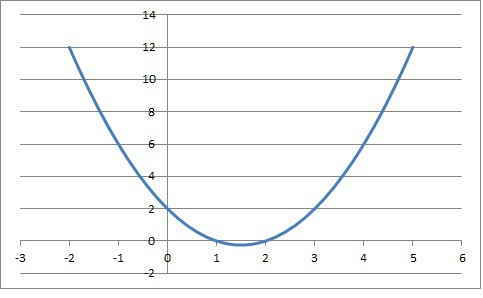This is the graph of the parabola from the quadratic y = x2 – 3x + 2. Note the zeros at x = 1 and x = 2, and the vertex (lowest point) at x = 1.5, halfway between the two zeros.

#### Example 2: One Repeated Real Solution (Zero Discriminant)

Consider the quadratic equation x2 + 4x + 4 = 0.  This equation is already in quadratic standard form.

We have coefficient values of a = 1, b = 4, and c = 4.

Remember that the quadratic formula is

Substituting into the quadratic formula, we get

After simplifying, we can reduce this to (-4 +/- 0) / 2, or x = -2.  This quadratic equation has one repeated real solution (which happens to be a negative integer).

The graph of this quadratic (a parabola) has a zero at x = -2.  In this case, the zero is also the vertex (since the zero is a double root).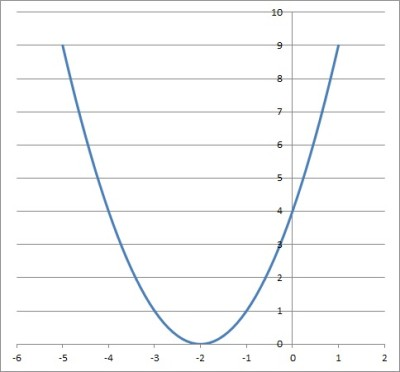This is the graph of the parabola from the quadratic y = x2 + 4x + 4. Note the zero at x = -2, which is also the vertex (lowest point), since x = -2 is a repeated real root.

#### Example 3: Two Complex Conjugate Solutions (Negative Discriminant)

Consider the quadratic equation x2 + x + 1 = 0.  This equation is already in quadratic standard form.

We have coefficient values of a = 1, b = 1, and c = 1.

Remember that the quadratic formula is

Substituting into the quadratic formula, we get

After simplifying, we can reduce this to [-1 +/- √(-3)] / 2, or x = (-1 + √3i)/2 and x = (-1 – √3i)/2.  This quadratic equation has two complex conjugate solutions.

The graph of this quadratic (a parabola) has no zeros.  The vertex is at x = -1 (found by taking the average of the two complex conjugate roots).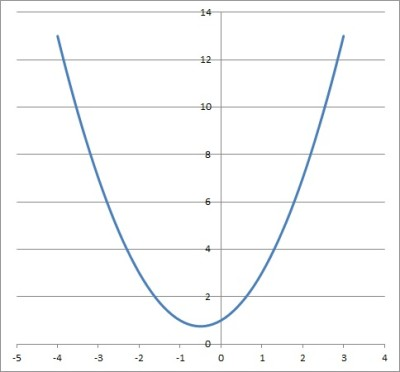This is the graph of the parabola from the quadratic y = x2 + x + 1. Note that there is no zero, since there are two complex conjugate roots. Thus, the graph never intersects the x-axis (y = 0).

### Can You Always Use The Quadratic Formula?

You can always use the quadratic formula to solve a quadratic equation.  This is true no matter the values of a, b, and c.

No matter how difficult it is to factor a quadratic, you can always use the quadratic formula to find solutions (roots) and then use those roots to find factors (more on this below).

### Does The Quadratic Formula Work For Complex Coefficients?

The quadratic formula works for complex coefficients.  Even if a, b, and c are pure imaginary numbers or complex numbers, the formula will still work.

### Can You Factor Using The Quadratic Formula?

You can factor using the quadratic formula.  After finding the roots r and s, we write the factors as (x – r) and (x – s).  We use the original value of a from the quadratic standard form ax2 + bx + c = 0 to factor the quadratic as:

• a(x – r)(x – s) = 0

Note that for real repeated root (zero discriminant), r = s and we get:

• a(x – r)(x – s) = 0
• a(x – s)(x – s) = 0
• a(x – s)2 = 0

#### Example 1: Factor By Using The Quadratic Formula (Two Distinct Real Roots)

Consider the quadratic equation x2 – 3x + 2 = 0.  This equation is already in quadratic standard form.

We have coefficient values of a = 1, b = -3, and c = 2.

We solved this above to get distinct real roots of r = 1 and s = 2.  So, our two factors are x – r and x – s, or x – 1 and x – 2.

Then the quadratic factors as a(x – r)(x – s) or 1(x – 1)(x – 2).

So x2 – 3x + 2 = (x – 1)(x – 2).

#### Example 2: Factor By Using The Quadratic Formula (One Repeated Real Root)

Consider the quadratic equation x2 + 4x + 4 = 0.  This equation is already in quadratic standard form.

We have coefficient values of a = 1, b = 4, and c = 4.

We solved this above to get a repeated real root of s = -2.  So, our factors are x – s and x – s, or x + 2 and x + 2.

Then the quadratic factors as a(x – s)(x – s) or 1(x + 2)(x + 2).

So x2 + 4x + 4 = (x + 2)(x + 2).

#### Example 3: Factor By Using The Quadratic Formula (Two Complex Conjugate Roots)

Consider the quadratic equation x2 + x + 1 = 0.  This equation is already in quadratic standard form.

We have coefficient values of a = 1, b = 1, and c = 1.

We solved this above to get distinct real roots of r = (-1 + √3i)/2 and s = (-1 – √3i)/2.  So, our two factors are x – r and x – s, or x – ((-1 + √3i)/2) and x – ((-1 – √3i)/2).

Then the quadratic factors as a(x – r)(x – s) or 1(x – ((-1 + √3i)/2))( x – ((-1 – √3i)/2)).

So x2 + x + 1 = (x + 0.5 + √3i/2))(x + 0.5 – √3i/2).

### Can You Use The Quadratic Formula For x3 (Does Quadratic Formula Work For Cubic?)

You cannot use the quadratic formula for x3 (a cubic equation).  However, there is a formula to solve a cubic – you can learn more here.

### Can You Use The Quadratic Formula For x4?

In certain cases, we can use the quadratic formula for a quartic equation with an x4 term.  This is done by a change of variables to t = x2 when we have a quartic equation of the form ax4 + bx2 + c = 0.

Let’s look at an example of how this works.

#### Example: Using The Quadratic Formula To Solve A Special Quartic

Consider the quartic equation x4 – 5x2 + 4 = 0. Its graph is below: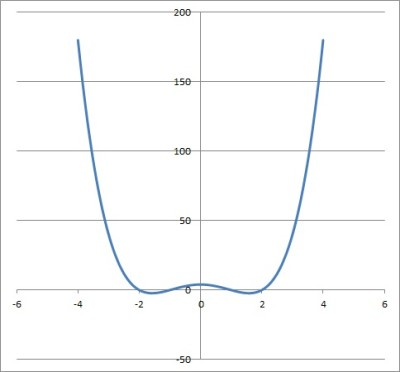This is the graph of the quartic y = x4 – 5x2 + 4. Note the zeros at x = -2, x = -1, x = 1, and x = 2.

First, we make the change of variables t = x2 to get:

• t2 – 5t + 4 = 0

which is a quadratic equation.  We can solve this easily with the quadratic formula, using a = 1, b = -5, and c = 4.

It is also easy to see that we can factor this quadratic as:

• (t – 1)(t – 4) = 0

which gives us solutions of t = 1 and t = 4.

Going back to the “x” variable, we get x2 = 1 and x2 = 4.

Taking square roots (both positive and negative) for both equations gives us four possible solutions: x = -1, x = 1, x = -2, and x = 2.

So, we can factor the original quartic equation as:

• x4 – 5x2 + 4 = (x – 1)(x + 1)(x – 2)(x + 2)

### How To Derive The Quadratic Formula (Prove The Quadratic Formula)

Here are the basic steps to derive the quadratic formula:

• First, begin with a quadratic equation in standard form, ax2 + bx + c = 0.
• Next, divide both sides by a to get a monic polynomial (with a leading coefficient of 1): x2 + (b/a)x + (c/a) = 0.
• Then, replace b/a by B and c/a by C to make your calculations easier: x2 + Bx + C = 0.
• Now, subtract C from both sides: x2 + Bx = -C.
• Next, add B2 / 4 to both sides (this is the “completing the square” step): x2 + Bx + (B2/4) = -C + (B2/4).
• Then, factor the left side as (x + B/2)2: (x + B/2)2 = -C + (B2/4)
• Now, take the square root of both sides. (x + B/2) = √(-C + (B2/4))
• Next, subtract B/2 from both sides to get the solutions of the quadratic equation.
• Finally, replace B by b/a and C by c/a, then simplify to get the quadratic formula.

You can learn how to complete the square to solve a quadratic equation here.

## Conclusion

Now you know what the quadratic formula is, when to use it, and how to use it.  You also know about the three possible cases for the solutions and when each case occurs (depending on the sign of the discriminant).

I hope you found this article helpful.  If so, please share it with someone who can use the information.